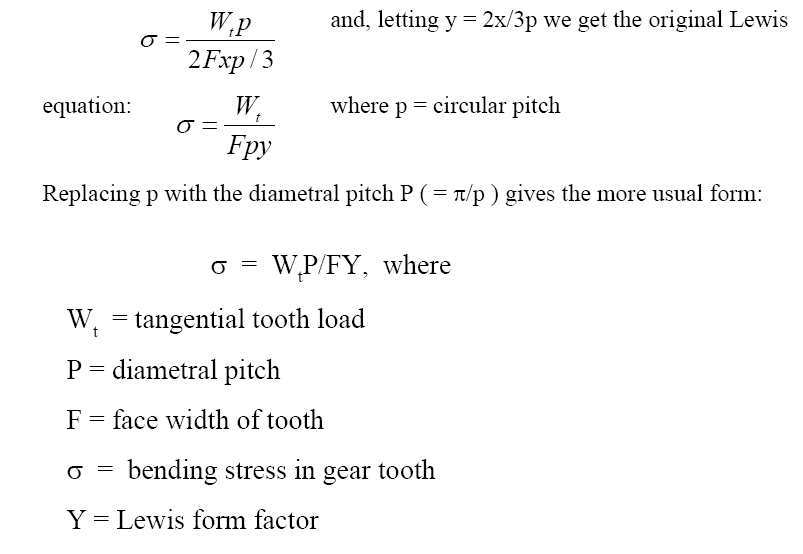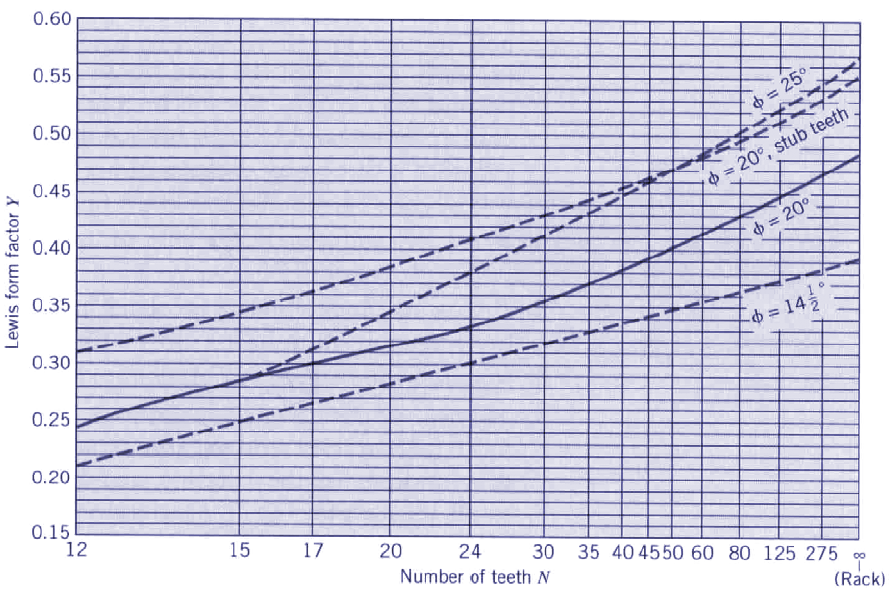Lewis Factor Equation and Calculator for Gear Tooth

Gear Products and Suppliers | Gear Knowledge Menu

The first equation used for the bending stress was the Lewis equation. This is derived by treating the tooth as a simple cantilever and with tooth contact occurring at the tip as shown above.ALL calculators require a Premium Membership

Only the tangent component (Wt) is considered. It is also assumed that only one pair of teeth is incontact. Stress concentrations at the tooth root fillet are ignored. It can be shown that the maximum bending stress occurs at the tangent points on the parabola shown above. Use of the standard equation for bending stress (σ= Mc/I) leads to:.Where:

Wt = (lbsf, N)
P = (in, mm)
F = (in, mm)
σ = (psi, Pa)
Y = DimensionlessRelated:© Copyright 2000 - 2019, by Engineers Edge, LLC www.engineersedge.comMembership Register | LoginHomeEngineering Book StoreEngineering ForumExcel App. DownloadsOnline Books & ManualsEngineering NewsEngineering VideosEngineering CalculatorsEngineering ToolboxGD&T Training Geometric Dimensioning TolerancingDFM DFA TrainingTraining Online EngineeringAdvertising CenterCopyright Notice